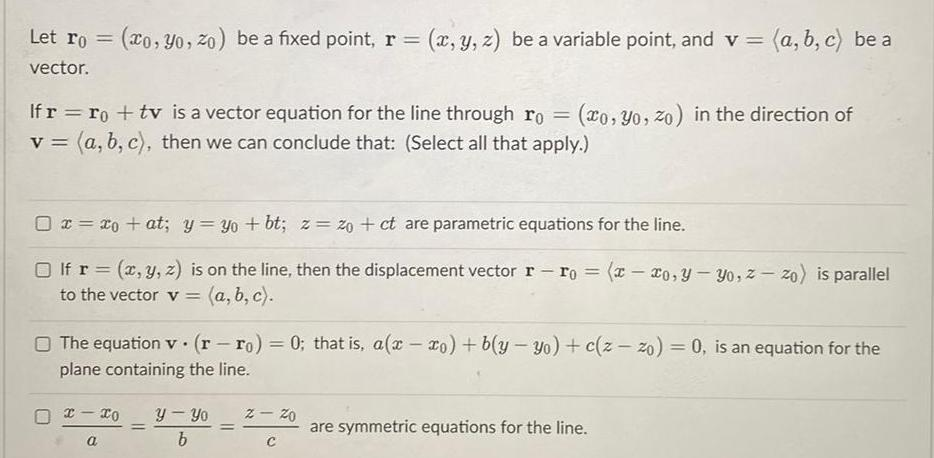Question:

# Let ro co yo zo be a fixed point r x y z be a variable point

Last updated: 8/16/2023Let ro co yo zo be a fixed point r x y z be a variable point and v a b c be a vector If r ro tv is a vector equation for the line through ro xo Yo zo in the direction of v a b c then we can conclude that Select all that apply 0 x x at y yo bt z 20 ct are parametric equations for the line If r x y z is on the line then the displacement vector r ro x xo y yo z zo is parallel a b c to the vector v The equation v rro 0 that is a x xo b y yo c z zo 0 is an equation for the plane containing the line x xo y Yo a b z 20 C are symmetric equations for the line